Name:    2nd Grade Numbers in Operations in Base Ten- Use place value understanding and properties of operations to add and subtract, Standard 7, Pre Test 1 Add and subtract within 1000, using concrete models or drawings and strategies based on place value, properties of operations, and/or the relationship between addition and subtraction; relate the strategy to a written method. Understand that in adding or subtracting three-digit numbers, one adds or subtracts hundreds and hundreds, tens and tens, ones and ones; and sometimes it is necessary to compose or decompose tens or hundreds.

Multiple Choice
Identify the choice that best completes the statement or answers the question.

1.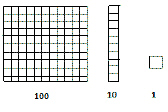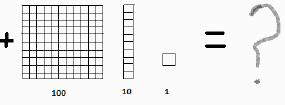Remember these drawings and what each one represents.
 a. 66 c. 6 b. 211 d. 222

2.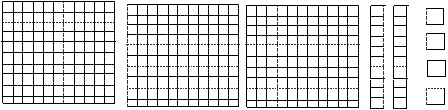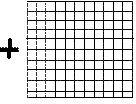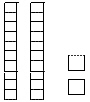a. 446 c. 460 b. 202 d. 422

3.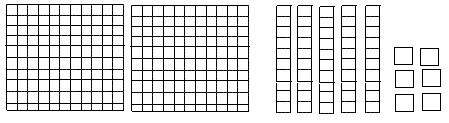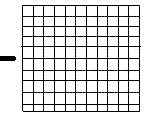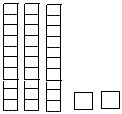a. 388 c. 195 b. 124 d. 194

4.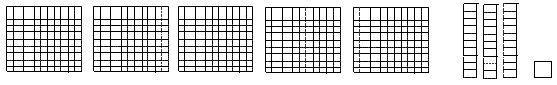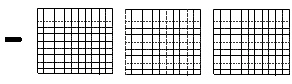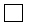_____________________________________________________________________

 a. 232 c. 230 b. 831 d. 832

5.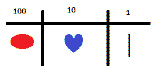Use these symbols in the next questions.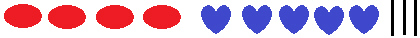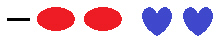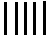__________________________________________________________

 a. 228 c. 230 b. 225 d. 678

6.Use these symbols for the problem below.__________________________________________________________

 a. 999 c. 1000 b. 1001 d. 990

7.Use these symbols in the problem below.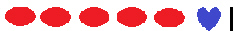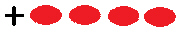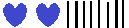__________________________________________________________

 a. 930 c. 199 b. 800 d. 940

8.Use these symbols in the problem below.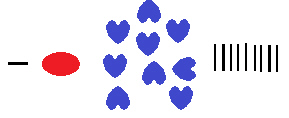__________________________________________________________

 a. 367 c. 641 b. 243 d. 222

9.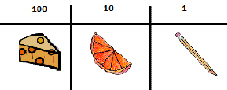Use the symbols to answer the next questions.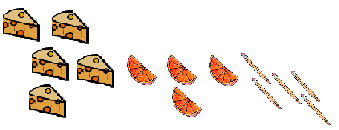__________________________________________________________

 a. 333 c. 211 b. 779 d. 222

10.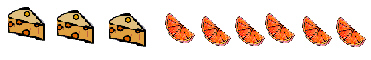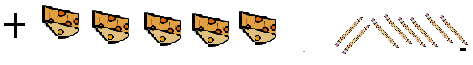__________________________________________________________

 a. 260 c. 251 b. 890 d. 868